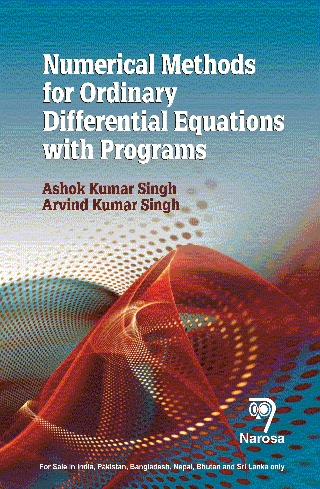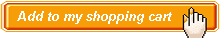Numerical Methods for Ordinary Differential Equations with Programs Authors:   Ashok Kumar Singh, Arvind Kumar Singh ISBN: 978-81-8487-617-8 Publication Year:   2018 Pages:   260 Binding:   Paper Back About the book Differential equations find its applications in all fields of science and engineering because it can describe the modeling of nearly all systems involving rate of change. Due to this fact, it has widespread use in physics, engineering, economics, social science and also in biology. Many systems involving differential equations are so complex, or the systems they describe are so large, that a purely mathematical analysis is not possible and it provides only the existence of the solution, therefore, we have to seek the approximate solution by means of the numerical methods. Hence in these types of complex systems, the computer simulations and numerical approximations are useful. The techniques for solving differential equations based on the numerical approximations can be used to handle the complex systems of differential equations on a common PC now-a-days. This is the first book in which the numerical solution procedures of six important methods are given for all the three types of the boundary conditions with programs in C. Key Features Table of content Preface / Numerical Methods for Initial Value Problems / Solution by Shooting Method / Solution by Finite Difference Method / Solution by Control Volume Method / Solution by Cubic Spline Method / Solution by Variational Methods / Solution by Finite Element Method / Appendices / References and Bibliography / Index.Audience Under – Post graduate Students, Teachers and Professionals